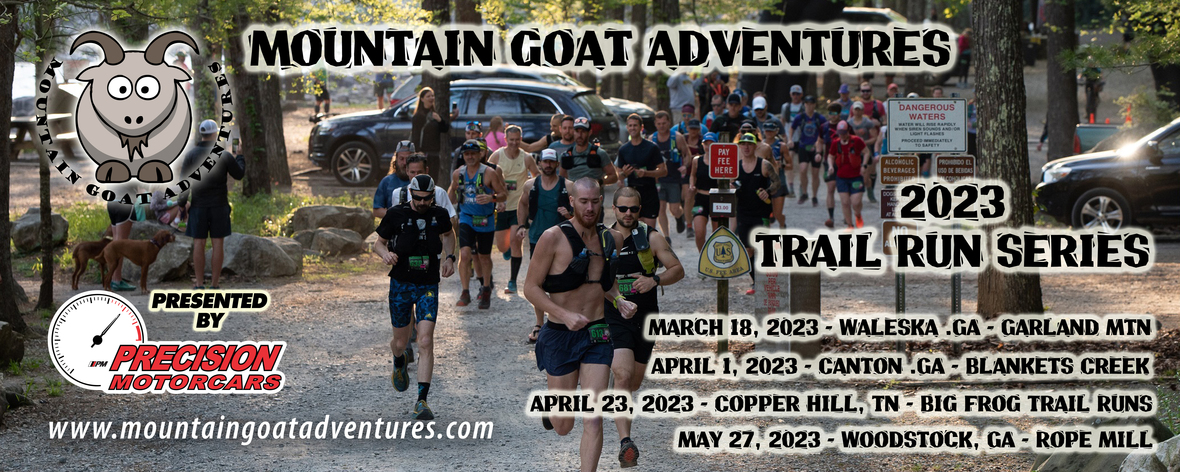# We have plenty of room left in this event as well as plenty of shirts and swag if you have friends who'd like to join us!

 table div table+table+table+table div table{width:100%;padding:0}table div table+table+table+table div table img{width:96.23%;padding:0;float:none}table div table+table+table+table div table td{width:100%;padding:0 1.88% 18px}/* styles */## Address of Ocoee Whitewater Center (RACE START): 4400 US-64, Copperhill, TN 37317

The race begins and ends at the Ocoee Whitewater Center. To enter the venue in the morning, you will need to actually drive past the first entrance to the Whitewater Center on US 64 and enter from the far end of the parking lot and drive back towards the bridge that goes over the river. Once you park, walk across the bridge and make a left towards the picnic shelter, which is where check-in will be.

It is \$3 per vehicle to park for the day, which can be paid at the USFS kiosks when you arrive or you can pay it online ahead of time (recommended).

If you are paying by cash, please bring exact change for the fee envelopes in the “iron ranger”. PLEASE NOTE THERE IS NO OVERNIGHT PARKING AT THE OCOEE WHITEWATER CENTER – SEE EVENT WEBSITE FOR CAMPING OPTIONS.

## Race Check-In: (2 Options)

Runners may check in on Saturday April 22nd from 4 pm to 7 pm, or on Sunday April 23rd from 6 am to 7:30 am. Race check-in will be at the Pavilion. You may have someone else check in for you and collect your race bib and swag ONLY if you send a signed waiver with them. You may have another racer pick up your swag for you if are unable to attend the race.

## Schedule:

7:40 am - Pre-race meeting for the Big Frog 50K near the pavilion
8:00 am - Big Frog 50K Race Start in Whitewater Center Parking Lot
8:10 am - Pre-race meeting for the 13.1 / 7 mile races near the pavilion
8:30 am - Big Frog 13.1 Start near pavilion
8:40 am - Big Frog 7 Mile Start near pavilion

Awards for each race will be presented near the finish line when the top 3 finishers in each category have crossed the finish line.

## Race Distance Changes:

If you want to switch your race distance, please do so by contacting me no later than Monday April 17th and we will get you taken care of.

## Courses and Aid Stations:

Please see the RACE COURSE MAPS page for these details.

## Miscellaneous Details:

• Course will be well marked with pink arrow signs at turns and pink flagging tape. We use A LOT of course markings and have an extremely low incidence of runners making wrong turns at our events.
* Please print race ACCIDENT WAIVER at home, sign and bring to race check-in with you.
• 50k runners are welcome to have support crews, however they may only meet and assist runners at aid stations and must not interfere with other runners on course. Crews may need to park a distance away from the aid stations and walk as parking is limited in some areas.
• Crew vehicles cannot block ANY roads and crews may not interfere with other runners on course or at aid stations.
• No pacers or other unregistered runners on course unless running with a minor
• 50k runners may send a "drop bag" that is one gallon-ish size to Boyd Gap (Mile 9) and Chestnut Mountain/FS 221 (Mile 20.3 and Mile 27.6). These need to be turned in at registration by 7:45 am and we will transport to the aid stations for you. Write your number BIG on the bag for identification purposes.
• Poles are allowed if you want to use them
• Dogs are allowed at the Ocoee Whitewater Center but they must be on a 6’ leash and you must be prepared to clean up after them
* 13.1 finisher medals will be available as you finish the race
* 50K finisher medals and hats will be available as you finish the race
• Post-Race water will be available for 7 and 13.1 mile runners.
• A post-race lunch with soda or water will be available for 50k runners as they finish.
* If you can no longer attend, you may sell your entry to someone else, but this needs to be done by 4/17. Payment is between buyer and seller..all I need is the new runner's name and email no later than 4/17. Sorry, no transfers to other events at this point as we've already ordered swag for you and the event is not full.

 table div table+table+table+table+table+table+table+table+table div table{width:100%;padding:0}table div table+table+table+table+table+table+table+table+table div table img{width:96.23%;padding:0;float:none}table div table+table+table+table+table+table+table+table+table div table td{width:100%;padding:0 1.88% 18px}/* styles */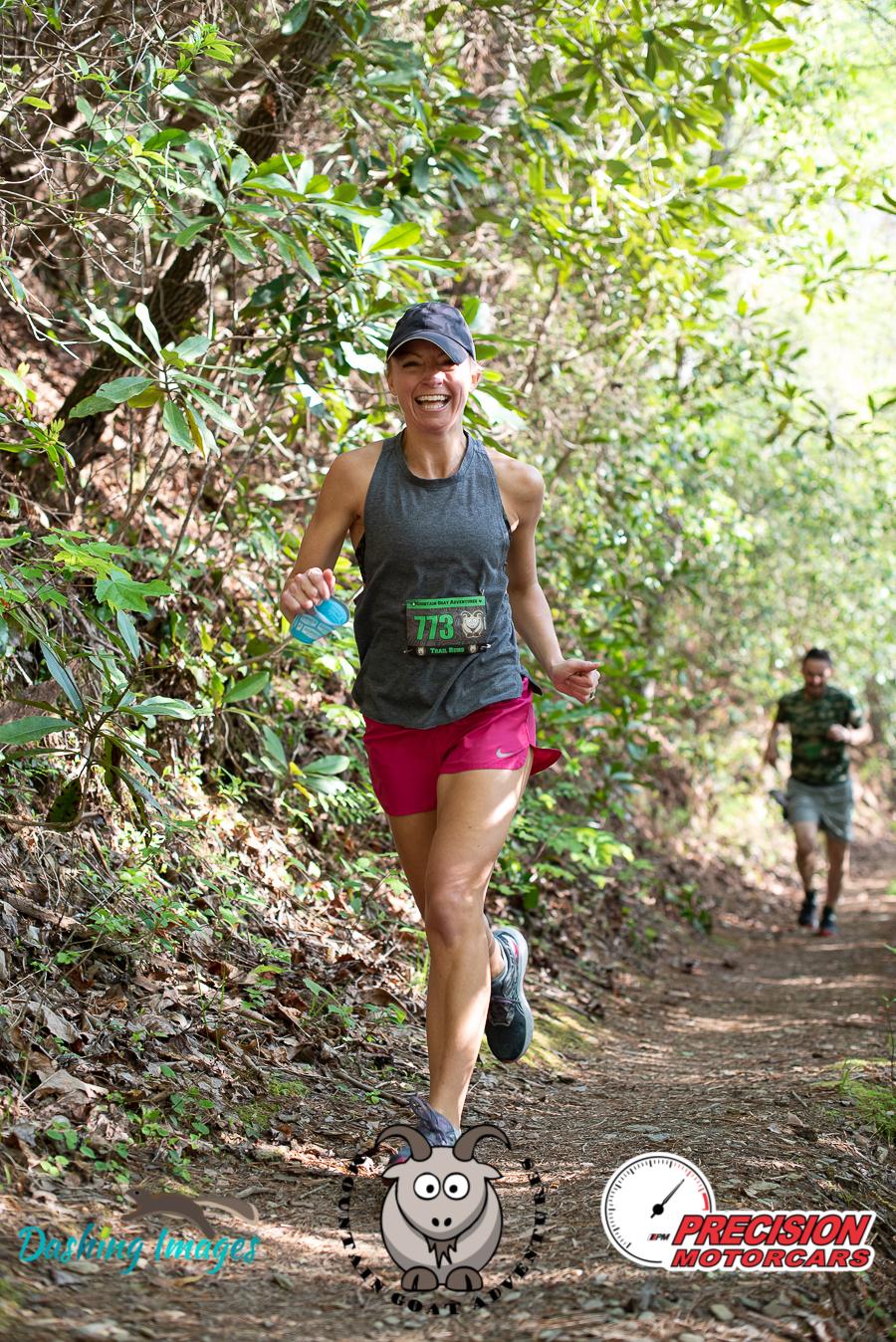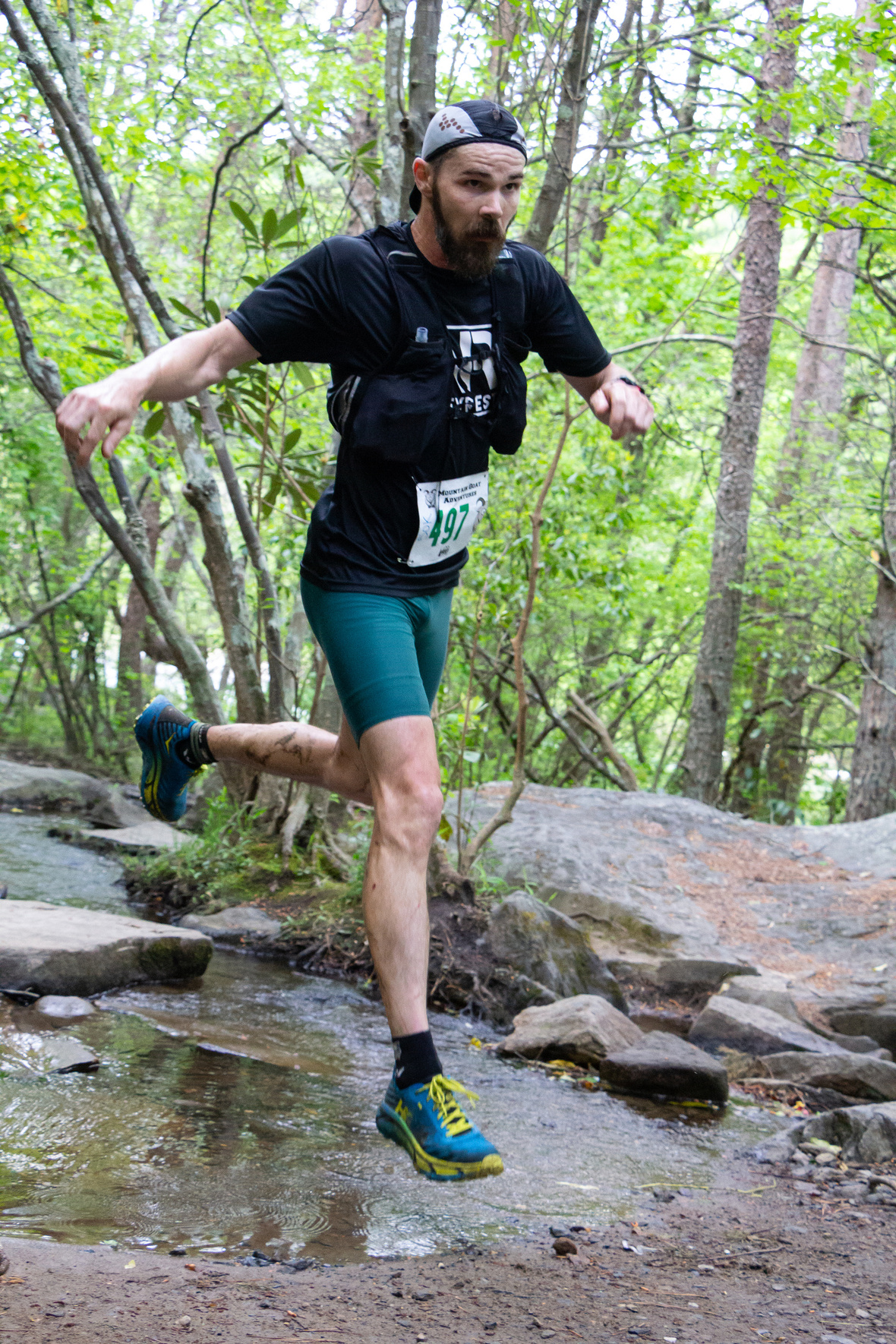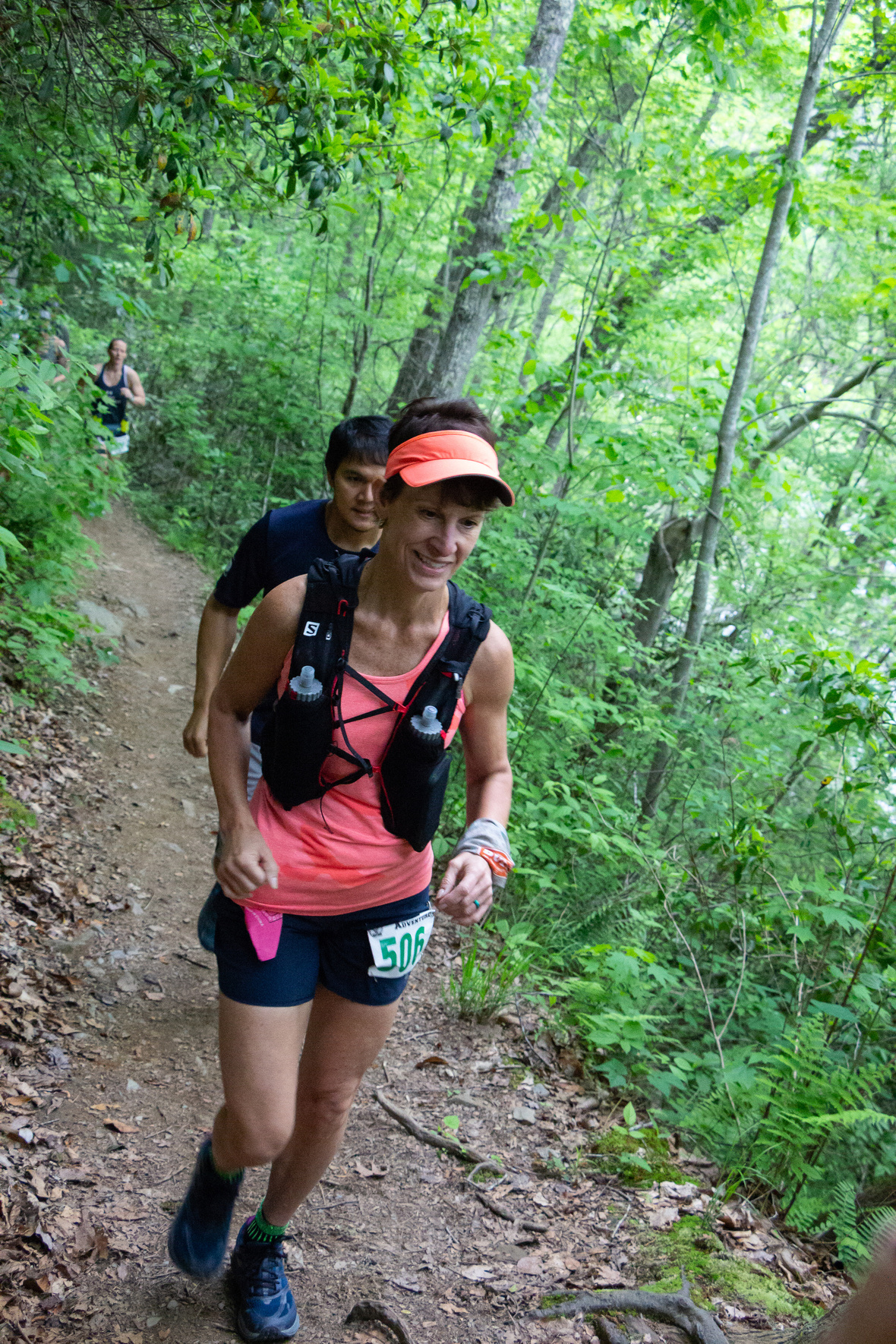table div table+table+table+table+table+table+table+table+table+table+table div table{width:100%;padding:0}table div table+table+table+table+table+table+table+table+table+table+table div table img{width:96.23%;padding:0;float:none}table div table+table+table+table+table+table+table+table+table+table+table div table td{width:100%;padding:0 1.88% 18px}/* styles */## Fueling Tips from Hammer Nutrition

 table div table+table+table+table+table+table+table+table+table+table+table+table+table div table,table.module-12{width:50.75%;float:right;padding:0}table div table+table+table+table+table+table+table+table+table+table+table+table+table div table a{border:0 none;text-decoration:none}table div table+table+table+table+table+table+table+table+table+table+table+table+table div table img{width:100%!important;border:0 none;text-decoration:none}table div table+table+table+table+table+table+table+table+table+table+table+table+table div table td{width:100%;padding:0 0 20px 20px}/* styles */ Your body needs to run at peak capacity so you can get your best result during the race. Here are a few tips from Hammer Nutrition to get started and make sure your body runs at peak capacity from start to finish! Tip #1 Hydration: Replenish, don’t replace! Keep fluid intake below 25 oz./hr during exercise. FIND OUT HOW MUCH Tip #2 Caloric Intake: Stay fueled, but not overwhelmed, by consuming 120-180 calories/hr. ARE CARBS BEST? Tip #3 Electrolytes: You need an array of vitamins and minerals, not just salt, to finish strong. WHAT DO YOU NEED? Tip #4 Pre-Event Fueling: Don’t tax your system with a meal within 3 hours of start time. BUT WHAT IF YOU'RE HUNGRY? Tip #5 Recovery: Refuel your tank with 60 minutes of finishing a workout. ARE CARBS OR PROTEIN BETTER?
 table div table+table+table+table+table+table+table+table+table+table+table+table+table+table div table{width:100%;padding:0}table div table+table+table+table+table+table+table+table+table+table+table+table+table+table div table img{width:96.23%;padding:0;float:none}table div table+table+table+table+table+table+table+table+table+table+table+table+table+table div table td{width:100%;padding:0 1.88% 18px}/* styles */## Visit Mulberry Gap Adventure Basecamp in Ellijay for Mountain Running and Amazing Meals!

 table div table+table+table+table+table+table+table+table+table+table+table+table+table+table+table+table div table td,table.module-15{width:100%;padding:0}table div table+table+table+table+table+table+table+table+table+table+table+table+table+table+table+table div table{width:100%;float:none;margin-left:auto;margin-right:auto;padding:0}table div table+table+table+table+table+table+table+table+table+table+table+table+table+table+table+table div table a{border:0 none;text-decoration:none}table div table+table+table+table+table+table+table+table+table+table+table+table+table+table+table+table div table img{width:100%!important;border:0 none;text-decoration:none}/* styles */
 table div table+table+table+table+table+table+table+table+table+table+table+table+table+table+table+table+table div table td,table.module-16{width:100%;padding:0}table div table+table+table+table+table+table+table+table+table+table+table+table+table+table+table+table+table div table{width:100%;float:none;margin-left:auto;margin-right:auto;padding:0}table div table+table+table+table+table+table+table+table+table+table+table+table+table+table+table+table+table div table a{border:0 none;text-decoration:none}table div table+table+table+table+table+table+table+table+table+table+table+table+table+table+table+table+table div table img{width:100%!important;border:0 none;text-decoration:none}/* styles */
 table div table+table+table+table+table+table+table+table+table+table+table+table+table+table+table+table+table+table div table{width:100%;padding:0}table div table+table+table+table+table+table+table+table+table+table+table+table+table+table+table+table+table+table div table img{width:96.23%;padding:0;float:none}table div table+table+table+table+table+table+table+table+table+table+table+table+table+table+table+table+table+table div table td{width:100%;padding:0 1.88% 18px}/* styles */## 2023 Full Event Calendar

 /* styles */ Want to check out our other events? Our 2023 calendar is below - we focus on mountain bike races, trail runs, and off-road duathlons. April 23, 2023 - Big Frog 50k - 13.1 mile - 7 mile Trail Runs May 27, 2023 - Rope Mill 10k and 13.1 mile Trail Run May 27, 2023 - Rope Mill Fast+Furious 15 MTB Race September 9, 2023 - Fools Gold 38 and 60 Mile MTB Races September 30, 2023 - Blankets Creek 3/6 Hour Race November 11, 2023 - Rope Mill Dirty Duathlon and 5k Trail Run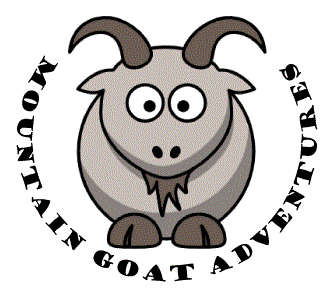## Thanks to our Trail Run Sponsors!

 table div table+table+table+table+table+table+table+table+table+table+table+table+table+table+table+table+table+table+table+table+table+table+table div table td,table.module-22{width:100%;padding:0}table div table+table+table+table+table+table+table+table+table+table+table+table+table+table+table+table+table+table+table+table+table+table+table div table{width:100%;float:none;margin-left:auto;margin-right:auto;padding:0}table div table+table+table+table+table+table+table+table+table+table+table+table+table+table+table+table+table+table+table+table+table+table+table div table a{border:0 none;text-decoration:none}table div table+table+table+table+table+table+table+table+table+table+table+table+table+table+table+table+table+table+table+table+table+table+table div table img{width:100%!important;border:0 none;text-decoration:none}/* styles */
 table div table+table+table+table+table+table+table+table+table+table+table+table+table+table+table+table+table+table+table+table+table+table+table+table div table td,table.module-23{width:100%;padding:0}table div table+table+table+table+table+table+table+table+table+table+table+table+table+table+table+table+table+table+table+table+table+table+table+table div table{width:100%;float:none;margin-left:auto;margin-right:auto;padding:0}table div table+table+table+table+table+table+table+table+table+table+table+table+table+table+table+table+table+table+table+table+table+table+table+table div table a{border:0 none;text-decoration:none}table div table+table+table+table+table+table+table+table+table+table+table+table+table+table+table+table+table+table+table+table+table+table+table+table div table img{width:100%!important;border:0 none;text-decoration:none}/* styles */
 table div table+table+table+table+table+table+table+table+table+table+table+table+table+table+table+table+table+table+table+table+table+table+table+table+table div table{width:100%;padding:0}table div table+table+table+table+table+table+table+table+table+table+table+table+table+table+table+table+table+table+table+table+table+table+table+table+table div table table{padding:0;float:left!important;width:61.509%!important}table div table+table+table+table+table+table+table+table+table+table+table+table+table+table+table+table+table+table+table+table+table+table+table+table+table div table table+table td,table div table+table+table+table+table+table+table+table+table+table+table+table+table+table+table+table+table+table+table+table+table+table+table+table+table div table td{padding-left:0;padding-right:0}table div table+table+table+table+table+table+table+table+table+table+table+table+table+table+table+table+table+table+table+table+table+table+table+table+table div table table td{padding-left:0;padding-right:20px}table div table+table+table+table+table+table+table+table+table+table+table+table+table+table+table+table+table+table+table+table+table+table+table+table+table div table table+table{float:left!important;width:38.491%!important}/* styles */
 table div table+table+table+table+table+table+table+table+table+table+table+table+table+table+table+table+table+table+table+table+table+table+table+table+table+table div table{width:100%;padding:0}table div table+table+table+table+table+table+table+table+table+table+table+table+table+table+table+table+table+table+table+table+table+table+table+table+table+table div table table{padding:0;float:left!important;width:61.32%!important}table div table+table+table+table+table+table+table+table+table+table+table+table+table+table+table+table+table+table+table+table+table+table+table+table+table+table div table table+table td,table div table+table+table+table+table+table+table+table+table+table+table+table+table+table+table+table+table+table+table+table+table+table+table+table+table+table div table td{padding-left:0;padding-right:0}table div table+table+table+table+table+table+table+table+table+table+table+table+table+table+table+table+table+table+table+table+table+table+table+table+table+table div table table td{padding-left:0;padding-right:20px}table div table+table+table+table+table+table+table+table+table+table+table+table+table+table+table+table+table+table+table+table+table+table+table+table+table+table div table table+table{float:left!important;width:38.68%!important}/* styles */
 table div table+table+table+table+table+table+table+table+table+table+table+table+table+table+table+table+table+table+table+table+table+table+table+table+table+table+table div table{width:100%;padding:0}table div table+table+table+table+table+table+table+table+table+table+table+table+table+table+table+table+table+table+table+table+table+table+table+table+table+table+table div table table{padding:0;float:left!important;width:56.415%!important}table div table+table+table+table+table+table+table+table+table+table+table+table+table+table+table+table+table+table+table+table+table+table+table+table+table+table+table div table table+table td,table div table+table+table+table+table+table+table+table+table+table+table+table+table+table+table+table+table+table+table+table+table+table+table+table+table+table+table div table td{padding-left:0;padding-right:0}table div table+table+table+table+table+table+table+table+table+table+table+table+table+table+table+table+table+table+table+table+table+table+table+table+table+table+table div table table td{padding-left:0;padding-right:20px}table div table+table+table+table+table+table+table+table+table+table+table+table+table+table+table+table+table+table+table+table+table+table+table+table+table+table+table div table table+table{float:left!important;width:43.585%!important}/* styles */
 table div table+table+table+table+table+table+table+table+table+table+table+table+table+table+table+table+table+table+table+table+table+table+table+table+table+table+table+table div table{width:100%;padding:0}table div table+table+table+table+table+table+table+table+table+table+table+table+table+table+table+table+table+table+table+table+table+table+table+table+table+table+table+table div table table{padding:0;float:left!important;width:41.886%!important}table div table+table+table+table+table+table+table+table+table+table+table+table+table+table+table+table+table+table+table+table+table+table+table+table+table+table+table+table div table table+table td,table div table+table+table+table+table+table+table+table+table+table+table+table+table+table+table+table+table+table+table+table+table+table+table+table+table+table+table+table div table td{padding-left:0;padding-right:0}table div table+table+table+table+table+table+table+table+table+table+table+table+table+table+table+table+table+table+table+table+table+table+table+table+table+table+table+table div table table td{padding-left:0;padding-right:20px}table div table+table+table+table+table+table+table+table+table+table+table+table+table+table+table+table+table+table+table+table+table+table+table+table+table+table+table+table div table table+table{float:left!important;width:58.114%!important}/* styles */
 table.module-28{width:55.85%;padding:0}table div table+table+table+table+table+table+table+table+table+table+table+table+table+table+table+table+table+table+table+table+table+table+table+table+table+table+table+table+table div table{width:55.85%;float:none;margin-left:auto;margin-right:auto;padding:0}table div table+table+table+table+table+table+table+table+table+table+table+table+table+table+table+table+table+table+table+table+table+table+table+table+table+table+table+table+table div table a{border:0 none;text-decoration:none}table div table+table+table+table+table+table+table+table+table+table+table+table+table+table+table+table+table+table+table+table+table+table+table+table+table+table+table+table+table div table img{width:100%!important;border:0 none;text-decoration:none}table div table+table+table+table+table+table+table+table+table+table+table+table+table+table+table+table+table+table+table+table+table+table+table+table+table+table+table+table+table div table td{width:100%;padding:0}/* styles */